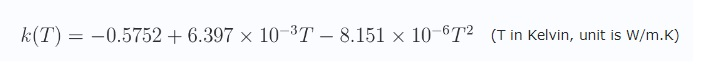## Fluids

•Devank12
Subscriber

I want to define thermal conductivity of  water that varies with temperature  I have  written a UDF based on the following polynomial equation.  I donot know it is right .If any correction plz inform me. Thanks#include "udf.h"

DEFINE_PROPERTY(knew,c,t)

{

real ktc;

real T = C_T(c,t);

ktc = – 0.5752 +(6.397*exp(– 3.0) * T ) – ( 8.151*exp(– 6.0) *pow(T,2.)) ;

C_UDMI(c,t,0) = ktc;

return ktc;

}

•DrAmine
Ansys Employee

Same as the other threads: you don't need an UDF as you can use the polynomial option there.